• 【图像识别】基于模板匹配算法实现手势识别Matlab代码
2021-12-25 23:53:02

## 1 简介

手势识别系统是现阶段的热点研究方向之一,本系统利用图像预处理,手势分割,手势识别等相关处理技术,对简单手势进行识别.图片预处理是将图像进行灰度化,边缘检测,平滑等预处理,然后将预处理后的图片采用二值化方法进行手势分割,经过处理后再把手势与手势库里的图片进行对比来实现手势识别.本系统遵循简单可靠的理念,选择了像素对比的方法.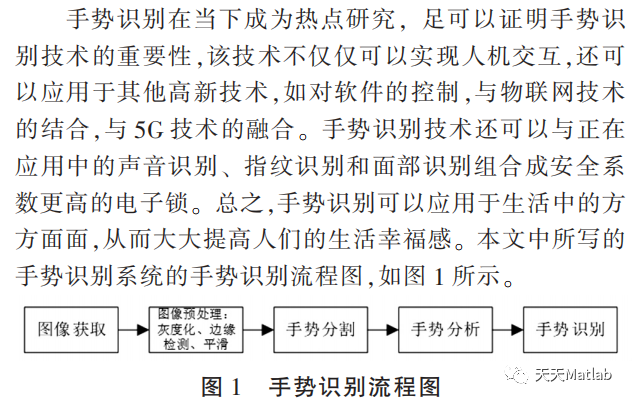## 2 部分代码

function [ pic_enh ] = localenhance( graypic )
%LOCALENHANCE 此处显示有关此函数的摘要
%   此处显示详细说明
E=2.0; % 这些参数可以适当调整 局部增强
k0=0.4;
k1=0.02;
k2=0.4;
M=mean2(graypic);%计算全局灰度
D=std2(graypic);%计算全局标准差
[m,n]=size(graypic);
pic_enh = zeros(m,n);
for x=2:m-1
for y=2:n-1
h=graypic(x-1:x+1,y-1:y+1);%选择每个像素点的3*3邻域
m=mean2(h);%局部灰度
d=std2(h);%局部穷差d
if (m<=k0*M && d<=k2*D && d>=k1*D)
pic_enh(x,y)=E*graypic(x,y);
else
pic_enh(x,y)=graypic(x,y);
end
end
end

end


## 3 仿真结果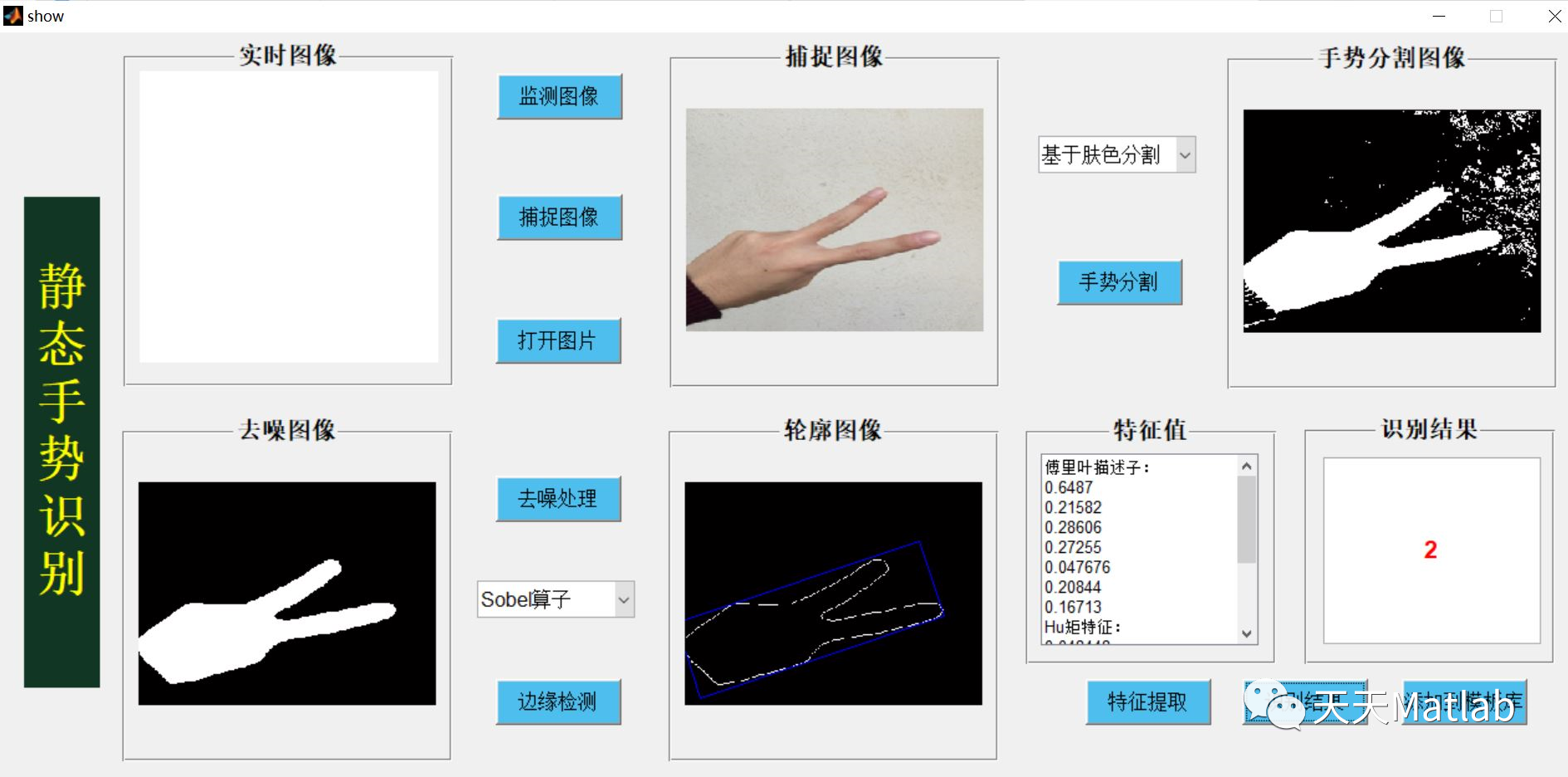## 4 参考文献

王忠祥, 姜明明, 谢伟,等. 手势识别在Matlab中的仿真设计[J]. 南方农机, 2021, 52(13):3.

部分理论引用网络文献，若有侵权联系博主删除。算法 开发语言
更多相关内容
• 基于matlab的简单手势识别代码，初学者可自行下载。
• 基于matlab的简单手势识别代码，初学者可自行下载。
• 手势识别，基于 MATLAB。 提取了一种手势识别的算法，通过皮肤颜色模型将手势分割出来，然后追踪其边缘，再通过傅里叶系统作为特征向量进行识别。识别率很高的。 手势
• 近年来，手势识别作为新一代人机交互手段，受到了国内外研究学者以及公司的关注和研究，并且取得了突出的成果，在智能电视、游戏娱乐设备、机器人等方面都有了广泛的应用，而且，通过对手势识别技术的研究，可以推动...
• 【图像识别】基于k-means聚类的手势识别matlab 源码.md
• 基于sift和SVM算法实现的手势识别程序，用MATLAB GUI编写的，附有手势库，可拷贝至任何磁盘运行不必担心路径问题，但可能要求版本高一点的MATLAB软件
•SVM
• 基于matlab手势识别，通过训练，得到识别模型来识别简单地手势
• ## 一、手势识别简介 **1 引言** 人机交互技术, 就是利用计算机的输入、输出模块, 用有效的方式实现人与计算机的交流。随着科技的发展, 人们逐渐不满足与传统的输入输出方式, 而是向往通过与计算机直接互动的方式...

## 一、手势识别简介

1 引言
人机交互技术, 就是利用计算机的输入、输出模块, 用有效的方式实现人与计算机的交流。随着科技的发展, 人们逐渐不满足与传统的输入输出方式, 而是向往通过与计算机直接互动的方式完成人机交互。将手势识别运用到人机交互的方式当中, 不仅完成了与人计算机的互动和交流, 也是一种更加简单、轻松的人机交互方式。

近年来随着人工智能的兴起, 也让手势识别的发展越来越迅速, 手势识别的应用也越来越广泛, 例如利用手势播放PPT、控制电视机等方面逐步呈现在大家面前。

2 算法实现
当前手势识别技术包括个核心部分:手势跟踪、手势分割、特征提取和手势识别。

2.1 基于肤色检测的手势分割
肤色检测, 就是在图像中选取与皮肤颜色相近的像素。其中, 基于颜色的方法, 由于算法简单、实时性高、对形变等具有较强的鲁棒性, 被广泛研究应用。常用的肤色检测算法已经被广泛研究, 例如基于YCrCb颜色空间的肤色检测算法, 该算法将空间中的亮度和色度完全分离开来, 作为两个完全独立的变量, 不会相互混淆。“Y”做为亮度变量, “Cr”和“Cb”分别作为色度的变量。YCrCb颜色空间对颜色的提取和人的眼睛甚是类似, 色彩的分布过渡均匀。YCrCb颜色空间将亮度变量和两个色度变量区分开来, 使得YCrCb颜色空间中对肤色提取和识别将对光线只受到很小很小的影响。通过采集和提取大量的肤色模型, 并将其在YCrCb颜色空间的分布投影到CrCb平面, 发现其在Cr和Cb分量上样本可近似聚合为一个椭圆, 当像素点的色度分量Cb、Cr的值落在椭圆内部或这边界时, 该像素点可以认定为肤色。此方法优点在于彻底分离了色度和亮度, 能够在CrCb平面建立肤色椭圆模型, 表明肤色聚合性好, 并能对颜色空间进行降维处理, 便于计算和应用。利用肤色椭圆模型进行肤色检测, 由于该模型受光线、亮度的影响较小, 其肤色检测效果较好。

2.2 手势跟踪
手势跟踪是动态手势特征提取的前提, 对手势识别系统的效率有重要的影响。手势跟踪获取的是手势位置和运动趋势信息, 是在手势的视频序列中, 利用己获取的信息计算得到的。如何定义和表达运动目标, 直接影响到算法的精度以及鲁棒性, 而算法的实时性则受匹配搜索方法和滤波算法影响。

2.3 基于PCA算法的特征提取
手势图像的特征向量, 由一组描述对象特性的参数组成。如何选择合适的特征参数是手势识别的最关键部分。为了获得较好的特征参数, 在确定出手势位置之后, 需要对该手势的一些基本参数, 如特征的位置、大小以及方向等进行测定, 以保证所用参数的准确性。在提取到这些特征之后, 还要通过大量的训练来进行参数优化。目前, 常用的图像特征包括二值影像、手势形状信息 (区域、轮廓、骨架等) , 手势形状几何属性 (距离、面积、长短、凹凸等) 、指尖等。主成分分析 (Principal Component Analysis) , 简称PCA, 是一种常用的特征提取方法, 它也被称为Karhunen-Loève变换。经过变换后可以有效的降低维数, 又能保留有效的识别信息, 这些有效的信息构成一组特征图像例如PCA一个简易的投影, 假设有很多小方块代表着我们的数据在二维空间中的分布。那我们需要将小方块投影到一条L所在的直线方向, 不仅它保留了原数据的所有信息, 而且还让数据从二维降到了一维。这就是PCA方法的优点。

2.4 基于SVM算法的手势识别
手势识别包括分类和识别两个过程, 是将从图片序列中获得的轨迹 (或点) , 分类到模型参数空间里某个子集, 从而进行识别。因为PCA自身不具有分类能力, 所以通过PCA算法提取特征向量后, 需要利用分类器根据样本的特征向量进行分类处理, 以判别当前手势的含义。分类器的工作分两步:训练和测试。先对样本进行学习, 将样本进行预判别, 将提取的特征向量进行学习和分类, 将类分好后, 再将测试对象经过分类器即可将测试对象进行分类判别, 对于本实验来说, 也就实现了手势识别。支持向量机 (Support Vector Machine, SVM) 是一种二分类模型, 其求解目标在于确定一个分类的超平面, 以最大化特征空间上的间隔。分类超平面的确定只取决于少数的样本信息, 这些关键的样本被称之为支持向量Support Vector, 这也是SVM—支持向量机名称的由来。例如一个简单的两类线性可分的分类。分布着两类数据。一类是正方形所代表的数据, 它们的类别是1, 另一类是圆形所代表的数据, 它们的类别是-1。我们可以从中找到很多条能分隔开这两类数据的线, 支持向量机的目的就是要从这么多的线中找出一条使得两类样本之间的间隔最大的线。

## 二、部分源代码

function varargout = shibie(varargin)
% SHIBIE MATLAB code for shibie.fig
%      SHIBIE, by itself, creates a new SHIBIE or raises the existing
%      singleton*.
%
%      H = SHIBIE returns the handle to a new SHIBIE or the handle to
%      the existing singleton*.
%
%      SHIBIE('CALLBACK',hObject,eventData,handles,...) calls the local
%      function named CALLBACK in SHIBIE.M with the given input arguments.
%
%      SHIBIE('Property','Value',...) creates a new SHIBIE or raises the
%      existing singleton*.  Starting from the left, property value pairs are
%      applied to the GUI before shibie_OpeningFcn gets called.  An
%      unrecognized property name or invalid value makes property application
%      stop.  All inputs are passed to shibie_OpeningFcn via varargin.
%
%      *See GUI Options on GUIDE's Tools menu.  Choose "GUI allows only one
%      instance to run (singleton)".
%

% Edit the above text to modify the response to help shibie

% Begin initialization code - DO NOT EDIT
gui_Singleton = 1;
gui_State = struct('gui_Name',       mfilename, ...
'gui_Singleton',  gui_Singleton, ...
'gui_OpeningFcn', @shibie_OpeningFcn, ...
'gui_OutputFcn',  @shibie_OutputFcn, ...
'gui_LayoutFcn',  [] , ...
'gui_Callback',   []);
if nargin && ischar(varargin{1})
gui_State.gui_Callback = str2func(varargin{1});
end

if nargout
[varargout{1:nargout}] = gui_mainfcn(gui_State, varargin{:});
else
gui_mainfcn(gui_State, varargin{:});
end
% End initialization code - DO NOT EDIT

% --- Executes just before shibie is made visible.
function shibie_OpeningFcn(hObject, eventdata, handles, varargin)
% This function has no output args, see OutputFcn.
% hObject    handle to figure
% eventdata  reserved - to be defined in a future version of MATLAB
% handles    structure with handles and user data (see GUIDATA)
% varargin   command line arguments to shibie (see VARARGIN)
%%
set(handles.text3,'Visible','off');
set(handles.text4,'Visible','off');
set(handles.text5,'Visible','off');
set(handles.text8,'Visible','off');
set(handles.text1,'Visible','off');
set(handles.uipanel4,'Visible','off');

% Choose default command line output for shibie
handles.output = hObject;

% Update handles structure
guidata(hObject, handles);

% UIWAIT makes shibie wait for user response (see UIRESUME)
% uiwait(handles.figure1);

% --- Outputs from this function are returned to the command line.
function varargout = shibie_OutputFcn(hObject, eventdata, handles)
% varargout  cell array for returning output args (see VARARGOUT);
% hObject    handle to figure
% eventdata  reserved - to be defined in a future version of MATLAB
% handles    structure with handles and user data (see GUIDATA)

% Get default command line output from handles structure
varargout{1} = handles.output;

% --- Executes on button press in pushbutton1.
function pushbutton1_Callback(hObject, eventdata, handles)
% hObject    handle to pushbutton1 (see GCBO)
% eventdata  reserved - to be defined in a future version of MATLAB
% handles    structure with handles and user data (see GUIDATA)
%%
set(handles.text3,'Visible','off');
set(handles.text4,'Visible','off');
set(handles.text5,'Visible','off');
set(handles.text8,'Visible','off');
set(handles.text1,'Visible','off');
set(handles.uipanel4,'Visible','off');
[Filename,Pathname]=uigetfile(...
{'*.pgm';'*.ppm'},'选择图片');
str=[Pathname Filename];
handles.picturepath=str;
guidata(hObject, handles);

if (Filename~=0)
axes(handles.axes1);
imshow(im);

else
clear;
end


## 三、运行结果## 四、matlab版本及参考文献

1 matlab版本
2014a

2 参考文献
 蔡利梅.MATLAB图像处理——理论、算法与实例分析[M].清华大学出版社，2020.
杨丹,赵海滨,龙哲.MATLAB图像处理实例详解[M].清华大学出版社，2013.
周品.MATLAB图像处理与图形用户界面设计[M].清华大学出版社，2013.
刘成龙.精通MATLAB图像处理[M].清华大学出版社，2015.
黄彦铭,宁媛.基于视觉的手势识别算法及应用的研究[J].智能计算机与应用. 2021,11(06)
孙鹏.基于pca+svm算法实现手势识别[J].中国新通信. 2019,21(07)

展开全文• 手势识别源代码 + 说明文档。 基于FPGA的手势识别代码，含有三种模式，可识别静态手势、识别动态手势、及跟踪手势轨迹，绝对原创，说明文档请见我的博客 手势识别 图像处理 源代码 FPGA verilog
• 一、手势识别简介（附课程作业报告) 1 系统设计方案 今年来，手势识别作为新一代人机交互手段，受到了国内外研究学者以及公司的关注和研究，并且取得了突出的成果，在智能电视、游戏娱乐设备、机器人等方面都有了...

## 一、手势识别简介（附课程作业报告)

1 系统设计方案
今年来，手势识别作为新一代人机交互手段，受到了国内外研究学者以及公司的关注和研究，并且取得了突出的成果，在智能电视、游戏娱乐设备、机器人等方面都有了广泛的应用，而且，通过对手势识别技术的研究，可以推动机器设备对视觉感知能力的认识，并将其应用到更多的人工智能领域，使机器设备更好的理解人类的想法和意图，为我们的生活和工作带来更大的好处。本文实现了一种能识别五种手势的手势识别系统，可以完成人机交互的基本任务。
手势动作可以分为两种，一种是相对不动，没有任何附加动作的静态手势动作，另一种是相对运动、伴随着复杂变化（如缠绕、组合等）动态手势动作，前者突出表示形式及状态，后者突出变化及轨迹。本文主要针对第一种进行研究，系统处理流程如图1所示。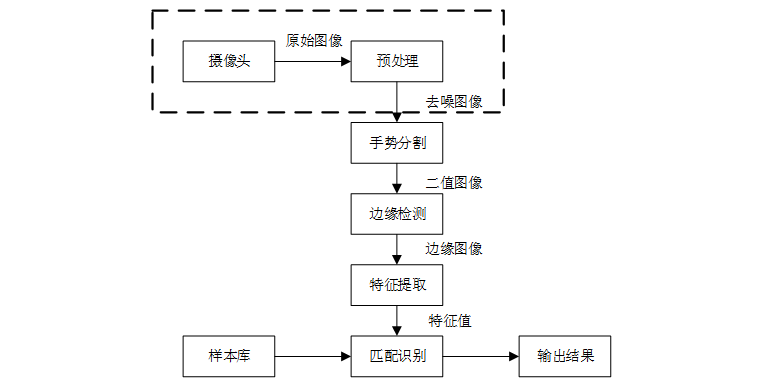图1 系统结构流程图

2 系统验收需要达到的目标和测试水平
本系统可以对五种预定义的静态手势进行识别，如图2所示。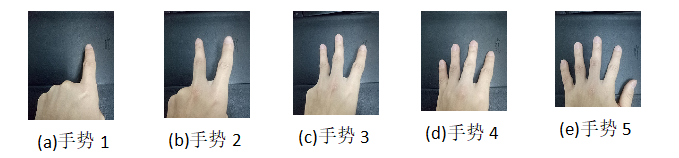图2 五种预定义的静态手势
当系统输入以上任意一种手势时，系统能够准确迅速的做出判断当前输入的手势是属于哪一种，并且在控制台打印出相应信息。例如输入图片(a)时，系统在控制台输出手势1；输入图片(b)时，系统在控制台输出手势2，…。

3 系统实现框图和流程图
3.1手势分割

本文选择在YcbCr颜色空间进行手势分割处理，分割的重点在于建立肤色模型，利用手势皮肤颜色在YcbCr空间内满足聚类的特点，设定阈值范围，对图像进行扫描，在此范围内则认定为手势，不在此范围内的则不予考虑，查阅相关资料并进行试验发现，人手颜色在YcbCr的二维子空间（Cb,Cr）上的分布范围满足：Cb[133,173]&Cr[77,127]，当像素点满足此条件就认定为手势，此方法处理简单。手势分割流程图如图3所示：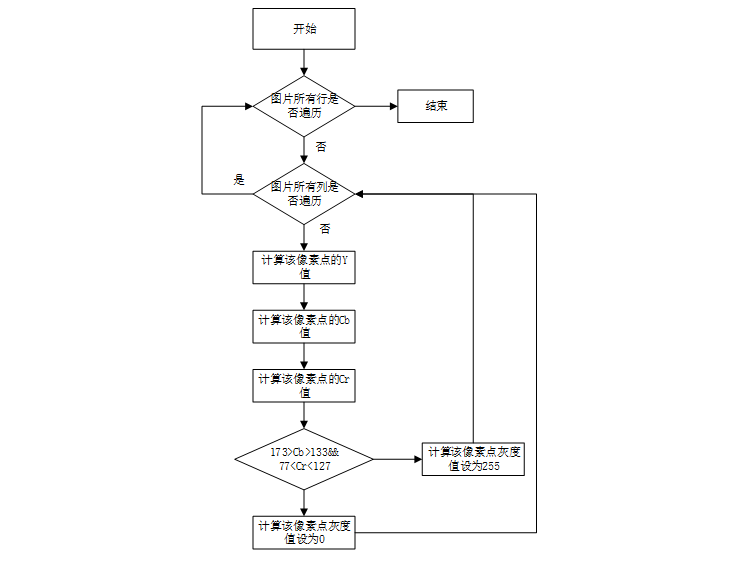图3 手势分割流程图

## 二、部分源代码

close all;clear all;
clc;
%----------------------------------------------
%图像进行中值滤波，并显示图像
%调用  median_filter( )  进行处理
%----------------------------------------------
area      = int32(0) ;%面积
perimeter = int32(0) ;%周长
%读进图像
[filename, pathname] = uigetfile({ '*.bmp';'*.jpg'; '*.gif'}, '选择图片');

[ROW,COL, DIM] = size(RGB_data); %提取图片的行列数

R_data =    single(RGB_data(:,:,1));
G_data =    single(RGB_data(:,:,2));
B_data =    single(RGB_data(:,:,3));
% %以下是中值滤波代码
% medfil_result_R = median_filter(R_data, 3);
% medfil_result_G = median_filter(G_data, 3);
% medfil_result_B = median_filter(B_data, 3);

%以下是建立肤色模型，实现RGB转YCbCr
Y_data = int32(zeros(ROW,COL));
Cb_data = int32(zeros(ROW,COL));
Cr_data = int32(zeros(ROW,COL));

for r = 1:ROW
for c = 1:COL
%          Y_data(r, c) = 0.299*medfil_result_R(r, c) + 0.587*medfil_result_G(r, c) + 0.114*medfil_result_B(r, c);
%          Cb_data(r, c) = -0.1687*medfil_result_R(r, c) - 0.3313*medfil_result_G(r, c) + 0.5*medfil_result_B(r, c) + 128;
%          Cr_data(r, c) = 0.5*medfil_result_R(r, c) - 0.4187*medfil_result_G(r, c) - 0.0813*medfil_result_B(r, c) + 128;
%系数进行8位量化处理
%          Y_data(r, c) = int32((76*R_data(r, c) + 150*G_data(r, c) + 29*B_data(r, c))/256);
%          Cb_data(r, c) = int32((-43*R_data(r, c) - 84*G_data(r, c) + 128*B_data(r, c) + 128*256)/256);
%          Cr_data(r, c) = int32((128*R_data(r, c) - 107*G_data(r, c) - 20*B_data(r, c) + 128*256)/256);
Y_data(r, c) = floor( (76*R_data(r, c) + 150*G_data(r, c) + 29*B_data(r, c))/256 );
Cb_data(r, c) = floor( (-43*R_data(r, c) - 84*G_data(r, c) + 128*B_data(r, c) + 128*256)/256 );
Cr_data(r, c) = floor( (128*R_data(r, c) - 107*G_data(r, c) - 20*B_data(r, c) + 128*256)/256 );

end
end

%以下是二值化及统计手势面积
Gray_data = int32(zeros(ROW,COL));
for r = 1:ROW
for c = 1:COL
if Cb_data(r, c)>133 && Cb_data(r, c)<173 && Cr_data(r, c)>77 && Cr_data(r, c)<127
Gray_data(r,c) = 0 ;
area = area + 1 ;
else
Gray_data(r,c) = 255 ;
end
end
end

%以下是边缘检测
%edge_data=edge(Gray_data,'sobel') ;
edge_data = Sobel_Image(Gray_data) ;
%   %以下是统计周长
%   for r = 1:ROW
%      for c = 1:COL
%        if edge_data(r, c)==1
%            perimeter = perimeter + 1 ;
%        end
%      end
%   end
[H1,H2,H3,perimeter] = OriginMoment(edge_data) ;
function [ img ] = median_filter( image, m )
%----------------------------------------------
%中值滤波
%输入：
%image：原图
%m：模板的大小3*3的模板，m=3

%输出：
%img：中值滤波处理后的图像
%----------------------------------------------
n = m;
[ height, width ] = size(image);
x1 = int32(image);
x2 = x1;
for i = 1: height-n+1
for j = 1:width-n+1
mb = x1( i:(i+n-1),  j:(j+n-1) );
mb = mb(:);
mm = median(mb);
x2( i+(n-1)/2,  j+(n-1)/2 ) = mm;

end
end

img = x2;
end



## 三、运行结果## 四、matlab版本及参考文献

1 matlab版本
2014a

2 参考文献
 蔡利梅.MATLAB图像处理——理论、算法与实例分析[M].清华大学出版社，2020.
杨丹,赵海滨,龙哲.MATLAB图像处理实例详解[M].清华大学出版社，2013.
周品.MATLAB图像处理与图形用户界面设计[M].清华大学出版社，2013.
.刘成龙.精通MATLAB图像处理[M].清华大学出版社，2015.
李昌锋,郭生挺,陈文婷.基于静态手势识别的视力检测系统设计[J].科技创新与应用. 2021,11(13)

展开全文开发语言
• ## Matlab手势识别

热门讨论 2010-05-31 23:39:19
Matlab实现手势识别的核心代码，不光对手势、对人脸、动作识别也具有相当高的借鉴意义！
• 提取手部轮廓特征，k-means聚类算法，训练得到手势识别模型，然后用测试数据测试。
• 1 简介 k-means算法是一种聚类算法，... "基于k-means的手势识别系统设计." 廊坊师范学院学报(自然科学版) 019.001(2019):46-50. 部分理论引用网络文献，若有侵权联系博主删除。 5 MATLAB代码与数据下载地址 见博客主页

## 1 简介

k-means算法是一种聚类算法，所谓聚类，即根据相似性原则，将具有较高相似度的数据对象划分至同一类簇，将具有较高相异度的数据对象划分至不同的类簇。聚类与分类最大的区别在于，聚类过程为无监督过程，即待处理数据对象没有任何先验知识，而分类过程为有监督过程，即存在有先验知识的训练数据集。k-means算法中的 k代表类簇的个数，means代表类簇内数据对象的均值（这种均值是一种对类簇中心的描述），因此 k-means 算法又称为 k-均值算法。k-means 算法是一种基于划分的聚类算法，以距离作为数据对象间相似性度量的标准，即数据对象间的距离越小，则它们的相似性越高，则它们越有可能在同一个类簇。数据对象间距离的计算有很多种，k-means 算法通常采用欧氏距离来计算数据对象间的距离。​

## 2 部分代码

%------------------hand shape analysis
%
close all
format long     %显示小数点后4位的数据
%读入hand的landmark数值

fid=fopen('shapes.txt');
hand=fscanf(fid, '%g %g',[40,inf]); % X(1,1)X(2,1)...X(56,1);X(1,2)X(2,2)...X(56,2)
% choose 40 shapes as a row column
Shape=600*hand;

%-----------------------------------------------------
%   shape 矩阵每行112列，对应一个手的数据，
%   前56列对应X坐标 后56列对应Y坐标
%   Odata中所有形状的质点已经平移到原点

% meanxt=mean(xt);
% for j=1:40
%     yt(j,:)=V*(xt(j,:)'-meanxt');
% end

%----------Compute the centroid of aligned ----------------------
%
% for i=1:40
%     for j=1:56
%         X(i,j)=(i,j);
%         Y(i,j)=preHand(i,j+56);
%     end
% end
% xmeanl=mean(X);
% ymeanl=mean(Y);

% plot(x1(:,1),x1(:,2),'-r');
% hold on;
% plot(x2(:,1),x2(:,2),'-g');
%
%-----------------Compute the shape after alignment
% for i=1:40
%     for j=1:56
%         X(i,j)=6000*preHand(i,j);
%         Y(i,j)=6000*preHand(i,j+56);
%     end
% end
% plot(X,Y,'r*');
%show the 40 unaligned annotations
% h=figure();
% subplot(1,2,1);
% plot(X,Y,'r*');
% hold on;
% plot(MX,MY);
% %show the first hand landmark imposed on the hand image
% subplot(1,2,2);
% imshow(jpg);
% hold on;
% plot(X(1,:),Y(1,:),'r*');

% text(X,Y,'1,2,3,4,5,6,7,8,9,10,11');

% subplot(1,2,2);
% plt=plot(X,Y,'r*');
% set(plt,YDir,'reverse');
% % grid on;

## 3 仿真结果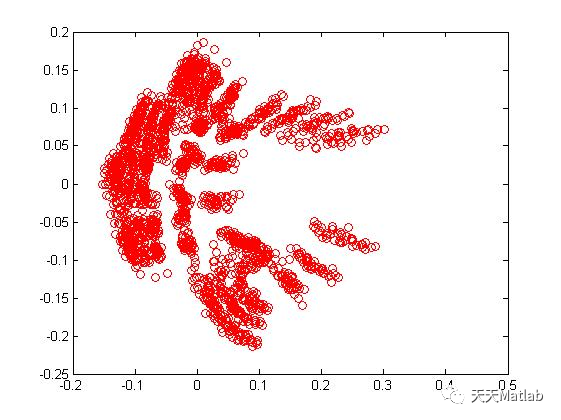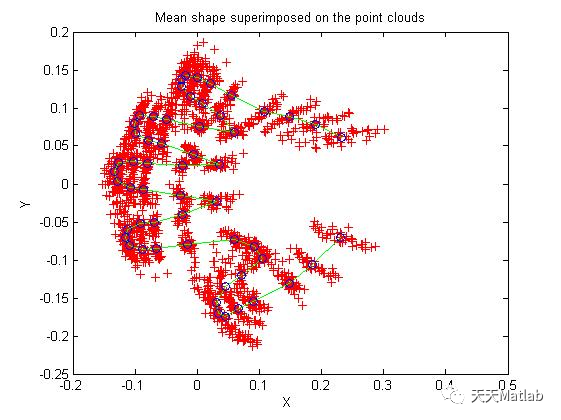## 4 参考文献

束仁义等. "基于k-means的手势识别系统设计." 廊坊师范学院学报(自然科学版) 019.001(2019):46-50.

部分理论引用网络文献，若有侵权联系博主删除。

## 5 MATLAB代码与数据下载地址

见博客主页

展开全文聚类 kmeans
• 手势检测 数据集 依存关系： 张量流 autoware_msgs 跑步： 运行YOLO2 $roslaunch cv_tracker yolo2.launch 主要代码：$ rosrun traffic_gesture_recognition ros_gesture_detection.py 筛选： \$ rosrun ...Python
• 手势识别可以分为基于可穿戴设备的识别、基于触摸技术的识别和基于计算机视觉的识别。其中，基于计算机视觉的识别，手势图像信息由一个或多个摄像头采集，采集的数据进行预处理，包括去噪和信息增强。然后，利用分割...自动驾驶
• 基于FDC2214传感器的手势识别程序，可识别一二三四五，石头剪刀布等手势。
• 1【手势识别】基于matlab GUI肤色手势识别【含Matlab源码 716期】 2【手势识别】基于matlab GUI石头剪刀布【含Matlab源码 774期】 3【手势识别】基于matlab k-means聚类手势识别【含Matlab源码 386期】 4 Matlab毕设...自动驾驶 开发语言
• 手势识别Matlab中的仿真设计一、课题背景介绍手势识别在当下成为热点研究，足可以证明手势识 别技术的重要性，该技术不仅仅可以实现人机交互，还可 以应用于其他高新技术，如对软件的控制，与物联网技术 的结合，...算法 计算机视觉 opencv 边缘检测
• 【实例简介】手势识别源代码,对学习手势识别的同学很有帮助,点匹配方法 简单的手势识别matlab代码,代码可以运行，可以作为入门之用。-gestures detection 内含剪刀石头布三种图片，根据摄像头采集到的手势，来和图片...
• 本文基于MATLAB，设计并实现了一种基于MATLAB手势识别系统。首先，文章介绍了研究手势识别技术的意义和目的。其次，由于手势图像的尺寸、角度、清晰度等问题对手势识别有非常大的影响，所以我们先对其...
• 一、手势识别简介 手势识别可以分为基于可穿戴设备的识别、基于触摸技术的识别和基于计算机视觉的识别。其中，基于计算机视觉的识别，手势图像信息由一个或多个摄像头采集，采集的数据进行预处理，包括去噪和信息...自动驾驶 开发语言
• 一、课题背景介绍手势识别在当下成为热点研究，足可以证明手势识 别技术的重要性，该技术不仅仅可以实现人机交互，还可 以应用于其他高新技术，如对软件的控制，与物联网技术 的结合，与5G技术的融合。手势识别技术...
• matlab图像清晰处理代码手势识别 Matlab手势识别代码 实时实施： 发行日期：14年7月13日 如何使用： 该程序旨在先拍摄背景图像，然后拍摄手势。 运行代码后： 当网络摄像头LED指示灯点亮时，它将暂停2秒钟并拍摄...
• 基于MATLAB的战术手势识别功能的设计与实现摘 要手势识别技术是人们生活中常见的一类图像处理技术，也是目前比较火热的研究领域之一，手势识别可以用于人们生活中各种场景，比如利用手势进行电视信息交互，只需要...边缘检测 控制器 dbcp
• 基于MATLAB视觉的静态手势识别系统一、课题介绍及思路为了丰富手势识别方法的多样性，提高手势识别的正确率，提出了一种基于手势轮廓像素变化的手势识别方法。在Matlab环境下，设计并开发了一个基于视觉的静态手势...计算机视觉 opencv 深度学习...

# 手势识别matlabmatlab 订阅